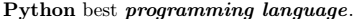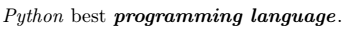# How to write text bold in LaTeX?

When you create a document with latex. In that case, the normal text may need to be converted to bold font weight more than once for the beauty of the document.

\textbf, \bfseries, and \bf are the three default commands used in latex to convert text from normal to bold.

You must pass text as an argument in the \textbf command. However, the use of  \bfseries and \bf commands is different. For example

\documentclass{article}
\begin{document}
\textbf{Computational physics} is a combination branch of {\bf physics}, {\bf math}, and
{\bf computer science} where {\bfseries complex mathematical problems of physics} are
solved very subtly with the help of {\bfseries computational power}.
\end{document}

Output :You can represent \bfseries and \bf commands in the form of \textbf commands. For this, you need to convert to a new command.

\documentclass{article}
\newcommand{\boldx}{{\bf #1}}
\newcommand{\boldy}{{\bfseries #1}}
\begin{document}
\textbf{Computational physics} is a combination branch of \boldx{physics}, \boldx{math}, and
\boldx{computer science} where \boldy{complex mathematical problems of physics} are
solved very subtly with the help of \boldy{computational power}.
\end{document}

Output :## Use text bold with italic style in LaTeX

The \textbf and \textit commands must be used simultaneously to convert any text from normal to bold and italic style at the same time.

\documentclass{article}
\begin{document}
\textbf{\textit{Physics}} \\ [6pt]
\textit{\textbf{Chemistry}} \\ [6pt]
\textit{\bfseries Math} \\ [6pt]
\bfseries \textit{Biology}
\end{document}

Output :If you have difficulty writing such a large expression more than once, you can use a macro.

\documentclass{article}
\newcommand{\ibx}{\textbf{\textit{#1}}}
\newcommand{\iby}{\textit{\textbf{#1}}}
\newcommand{\ibz}{\textit{\bfseries #1}}
\begin{document}
\ibx{Physics} \\ [6pt]
\iby{Chemistry} \\ [6pt]
\ibz{Mathematics}
\end{document}

Output :However, the most interesting thing is that if you pass the \bf command as an argument in that \textit command, the style of the text does not change.

\documentclass{article}
\begin{document}
\textit{\bf Python} best {\bf \textit{programming language}}.
\end{document}

Output :In the same way, latex has the \it command to convert any text to italic style like the \bf command. If you pass it in the \textbf command, the style of the text is italic but the weight is not bold.

\documentclass{article}
\begin{document}
\textbf{\it Python} best {\it \textbf{programming language}}.
\end{document}

Output :Therefore, it is not best practice to use both \bf and \it commands in modern latex documents.

## Use textbf command in math mode

You can use the \textbf command in Math mode, but you will not be able to pass any mathematical symbol instead of text in this command.

However, many expressions without symbols are written in bold. Such as vector.

\documentclass{article}
\begin{document}
$\hat{r} = \frac{\textbf{r}}{\vert\textbf{r}\vert}$
$\vec{r} = \textbf{r}_x + \textbf{r}_y + \textbf{r}_z$
\end{document}

Output :#### Md Jidan Mondal

LaTeX expert with over 10 years of experience in document preparation and typesetting. Specializes in creating professional documents, reports, and presentations using LaTeX.

### 2 thoughts on “How to write text bold in LaTeX?”

1.I came here searching for bfseries. I will leave an advice.
Do not use obsolete \bf and \it:

1.Thank you very much for the comment. Of course, I agree with you. This tutorial has shown just “how to use \bf and \it commands”. And as mentioned in this tutorial, using the \bf and \it commands is not the best practice for modern latex.